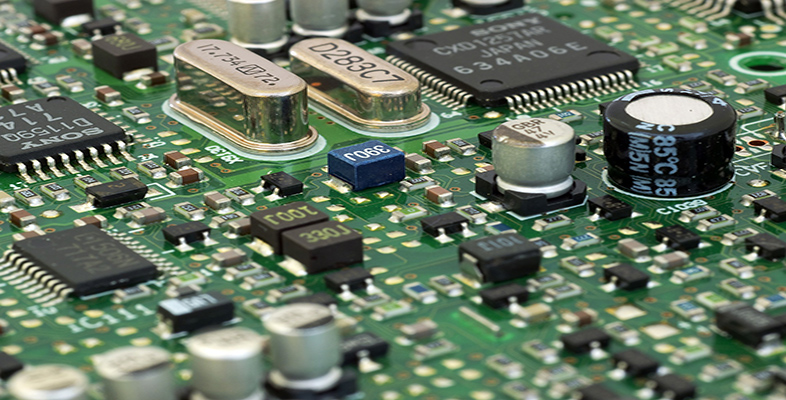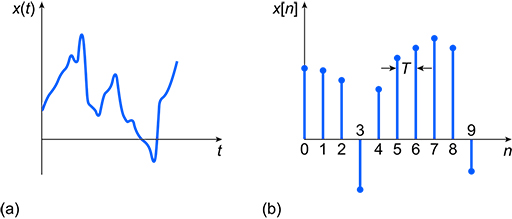Science, Maths & Technology

### Become an OU studentElectronic applications

Start this free course now. Just create an account and sign in. Enrol and complete the course for a free statement of participation or digital badge if available.

# 3.2 Characteristics of discrete-time and continuous-time signals

A continuous-time signal is shown in Figure 15(a). The signal is continuous because it has a value at any instance of time – that is, for any value of , it is possible read a value of from the graph. Most signals in the real world are continuous in time. For example, if you were monitoring the temperature of a room, you would be able to take a measured value of temperature at any time.Figure 15 (a) Continuous-time signal; (b) discrete-time signal

A discrete-time signal (sometimes referred to as a time-discrete signal or simply a discrete signal) is shown in Figure 15(b). In the rest of this course the standard convention of drawing the vertical lines in a discrete-time signal with a round dot on the end will be used; these lines-with-dots are often called ‘lollipops’. The signal in Figure 15(b) is discrete because it only has a value at fixed points placed at discrete time intervals seconds apart along the -axis. is called the sampling interval. Values of can be found for the integer values of , such as , , etc., but there is no value for the signal at, say, . Thus represents the number of the sample.

It is hard to think of examples of real-world discrete-time signals, since most real-world signals are continuous; however, if you took the temperature reading of a room every day at the same time, the result would be a discrete-time signal. Most discrete-time signals come from sampling continuous-time signals to get them into a digitised form that can be processed by digital computers.

## Activity 6

State whether the following are discrete-time signals or continuous-time signals, giving a reason for each answer:

• a.the wind speed across the blades of a wind turbine
• b.the position of a robotic arm as it picks items from a conveyor belt
• c.the total distance travelled by the robotic arm each hour over a 24-hour period.